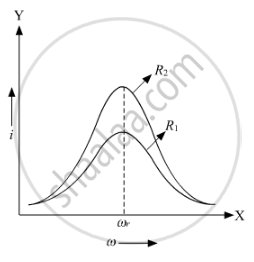Share

# Plot a Graph Showing Variation of Current 'I' as a Function of 'ω' for Two Resistances R1 and R2 (R1 > R2). - CBSE (Science) Class 12 - Physics

ConceptAc Voltage Applied to a Series Lcr Circuit

#### Question

A series LCR circuit is connected across an a.c. source of variable angular frequency 'ω'. Plot a graph showing variation of current 'i' as a function of 'ω' for two resistances R1 and R2 (R1 > R2).

Answer the following questions using this graph :

(a) In which case is the resonance sharper and why?

(b) In which case in the power dissipation more and why?

#### Solution

he variation of current with angular frequency for the two resistances R1 and R2 is shown in the graph below.Here,
i = Virtual current through the circuit
ω = Angular frequency of the source
ωr = Resonance frequency
From the graph, we can see that resonance for the resistance R2 is sharper than for R1 because resistance R2 is less than resistance R1. Therefore, at resonance, the value of peak current will rise more abruptly for a lower value of resistance.

b) Power associated with the resistance is given by

P=Ev Iv

where
Ev = Virtual voltage
Iv = Virtual current
From the graph, we can say that the virtual current in case of R2 is more than the virtual current in case of R1. Hence, the power dissipation in case of the circuit with R2 is more than that with R1.

Is there an error in this question or solution?

#### Video TutorialsVIEW ALL 

Solution Plot a Graph Showing Variation of Current 'I' as a Function of 'ω' for Two Resistances R1 and R2 (R1 > R2). Concept: Ac Voltage Applied to a Series Lcr Circuit.
S